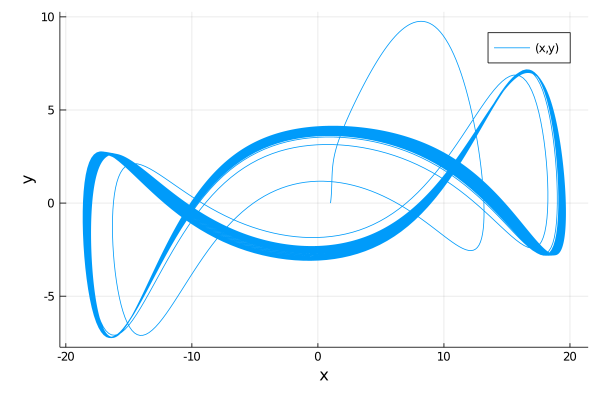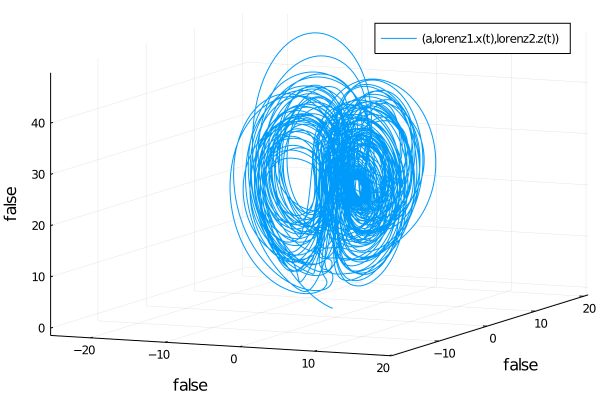{{ message }}

SciML / ModelingToolkit.jl Public

A modeling framework for automatically parallelized scientific machine learning (SciML) in Julia. A computer algebra system for integrated symbolics for physics-informed machine learning and automated transformations of differential equations

Switch branches/tags
Nothing to show

6e98586 2

Files

Failed to load latest commit information.
Type
Name
Commit time
Dec 3, 2021
Jan 3, 2022
Nov 9, 2021
Jan 15, 2022
Jan 15, 2022
Feb 27, 2018
Mar 19, 2021
Mar 10, 2021
Feb 28, 2021
Dec 23, 2021
Jan 15, 2022
Aug 16, 2021

ModelingToolkit.jl

ModelingToolkit.jl is a modeling framework for high-performance symbolic-numeric computation in scientific computing and scientific machine learning. It allows for users to give a high-level description of a model for symbolic preprocessing to analyze and enhance the model. ModelingToolkit can automatically generate fast functions for model components like Jacobians and Hessians, along with automatically sparsifying and parallelizing the computations. Automatic transformations, such as index reduction, can be applied to the model to make it easier for numerical solvers to handle.

For information on using the package, see the stable documentation. Use the in-development documentation for the version of the documentation which contains the unreleased features.

High-Level Examples

First, let's define a second order riff on the Lorenz equations, symbolically lower it to a first order system, symbolically generate the Jacobian function for the numerical integrator, and solve it.

using ModelingToolkit, OrdinaryDiffEq

@parameters t σ ρ β
@variables x(t) y(t) z(t)
D = Differential(t)

eqs = [D(D(x)) ~ σ*(y-x),
D(y) ~ x*-z)-y,
D(z) ~ x*y - β*z]

@named sys = ODESystem(eqs)
sys = ode_order_lowering(sys)

u0 = [D(x) => 2.0,
x => 1.0,
y => 0.0,
z => 0.0]

p  ==> 28.0,
ρ => 10.0,
β => 8/3]

tspan = (0.0,100.0)
prob = ODEProblem(sys,u0,tspan,p,jac=true)
sol = solve(prob,Tsit5())
using Plots; plot(sol,vars=(x,y))This automatically will have generated fast Jacobian functions, making it more optimized than directly building a function. In addition, we can then use ModelingToolkit to compose multiple ODE subsystems. Now, let's define two interacting Lorenz equations and simulate the resulting Differential-Algebraic Equation (DAE):

using ModelingToolkit, OrdinaryDiffEq

@parameters t σ ρ β
@variables x(t) y(t) z(t)
D = Differential(t)

eqs = [D(x) ~ σ*(y-x),
D(y) ~ x*-z)-y,
D(z) ~ x*y - β*z]

@named lorenz1 = ODESystem(eqs)
@named lorenz2 = ODESystem(eqs)

@variables a(t)
@parameters γ
connections = [0 ~ lorenz1.x + lorenz2.y + a*γ]
@named connected = ODESystem(connections,t,[a],[γ],systems=[lorenz1,lorenz2])

u0 = [lorenz1.x => 1.0,
lorenz1.y => 0.0,
lorenz1.z => 0.0,
lorenz2.x => 0.0,
lorenz2.y => 1.0,
lorenz2.z => 0.0,
a => 2.0]

p  = [lorenz1.σ => 10.0,
lorenz1.ρ => 28.0,
lorenz1.β => 8/3,
lorenz2.σ => 10.0,
lorenz2.ρ => 28.0,
lorenz2.β => 8/3,
γ => 2.0]

tspan = (0.0,100.0)
prob = ODEProblem(connected,u0,tspan,p)
sol = solve(prob,Rodas4())

using Plots; plot(sol,vars=(a,lorenz1.x,lorenz2.z))Citation

@misc{ma2021modelingtoolkit,
title={ModelingToolkit: A Composable Graph Transformation System For Equation-Based Modeling},
author={Yingbo Ma and Shashi Gowda and Ranjan Anantharaman and Chris Laughman and Viral Shah and Chris Rackauckas},
year={2021},
eprint={2103.05244},
archivePrefix={arXiv},
primaryClass={cs.MS}
}

A modeling framework for automatically parallelized scientific machine learning (SciML) in Julia. A computer algebra system for integrated symbolics for physics-informed machine learning and automated transformations of differential equations

v8.3.0 Latest
Jan 15, 2022